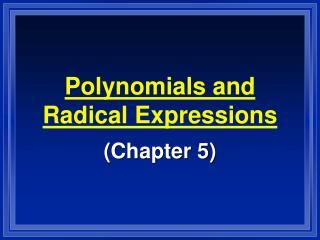DownloadDownload PresentationDownload Presentation- - - - - - - - - - - - - - - - - - - - - - - - - - - E N D - - - - - - - - - - - - - - - - - - - - - - - - - - -
##### Presentation Transcript

1. Polynomials and Radical Expressions (Chapter 5)

2. Properties of Exponents • am . an = a(m + n) • am /an = a(m  n) • (am)n = a(mn) • (a  b)m = am  bm • (a / b)m = am / bm • a0 = 1

3. Negative Exponents • a-n = 1 and 1 = an an a-n • Scientific Notation • a x 10n • where 1 < a < 10 and n is an integer.

4. Monomials (5.1) • monomial = an expression that is a number, a variable, or the product of a number and one or more variables. • constant = a monomial that contains no variables.

5. coefficient = the number part of a monomial. • degree (of a monomial) = the sum of the exponents of the variables. • 3 is a constant (degree of 0). • 3x2 has a degree of 2. • 3x2y4z5 has a degree of 11.

6. Variables in monomials must have whole number exponents. • If a variable is under a radical sign or in a denominator, then it is not a monomial. • and 1/x2are not monomials.

7. Polynomials (5.3) • polynomial = a monomial or a sum of monomials. • terms = the monomials that make up a polynomial. • like terms = terms with the same variables with the same exponents.

8. binomial = a polynomial with two unlike terms. • trinomial = a polynomial with three unlike terms. • degree (of a polynomial) = the degree of its largest monomial.

9. Adding Polynomials • To add polynomials, add like terms. • To subtract polynomials, subtract like terms, or • distribute the negative into the second polynomial and then add like terms.

10. Multiplying Polynomials • To multiply monomials, multiply the coefficients and follow the exponent rules for the variables. • To multiply two binomials together, use F.O.I.L. • To multiply larger polynomials, use M.E.T.W.E.T.

11. Dividing Polynomials (5.3) • To divide a polynomial by a monomial, divide each term by the monomial. • To divide a polynomial by another polynomial, use long division. • (D.M.S.B.R.)

12. To divide a polynomial by a first-degree binomial, use synthetic division. • Write the coefficients in a row (in descending order). • Write the opposite of the constant of the divisor to the left. • Bring down the first number.

13. Multiply the number with the constant. • Add the product with the next coefficient. • Repeat steps 4 and 5 until there are no more numbers. • Write the last answer as a remainder.

14. [If the divisor has a leading coefficient other than 1, that must be divided separately.] • Divide it out of the divisor first. • Do the synthetic division. • Then divide it out of the final answer.

15. Factoring (5.4) • factors (of a polynomial) = polynomials that divide evenly into a polynomial. • prime polynomials = polynomials that cannot be factored.

16. Types of Factoring: • Common factors • Difference of Squares • Difference of Cubes • Sum of Cubes • Perfect Square Trinomials • Guess and Check • Grouping (for 4 or more terms)

17. Graphs of Polynomial Functions (5.2) • A polynomial function cannot have: • Negative exponents on the variables • Variables in the denominator • Fractional exponents on the variables • Variables in a radicand

18. End Behavior of Polynomials • To determine the shape of a graph of a polynomial function, look at its leading coefficient and its degree. • PEHH – POLH – NELL – NOHL • (The x-intercepts and y-intercept will also be important in graphing polynomial functions.)